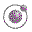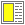Higher-order Functions - slide 24 : 34

Ad hoc currying in Scheme (1)
It is possible to achieve 'the currying effect' by generalizing functions, which requires two or more parameters, to only require a single parameter
Motivation:
 Expression Value `(map li (list "one" "two" "three"))` ```("
• one
• " "
• two
• " "
• three
• ")``` `(define li-mapper (map li))` map: expects at least 2 arguments, given 1 ```(define li-mapper ((curry2 map) li)) (li-mapper (list "one" "two" "three"))``` ```("
• one
• " "
• two
• " "
• three
• ")```The function curry-generalized.